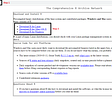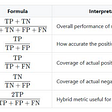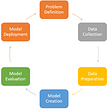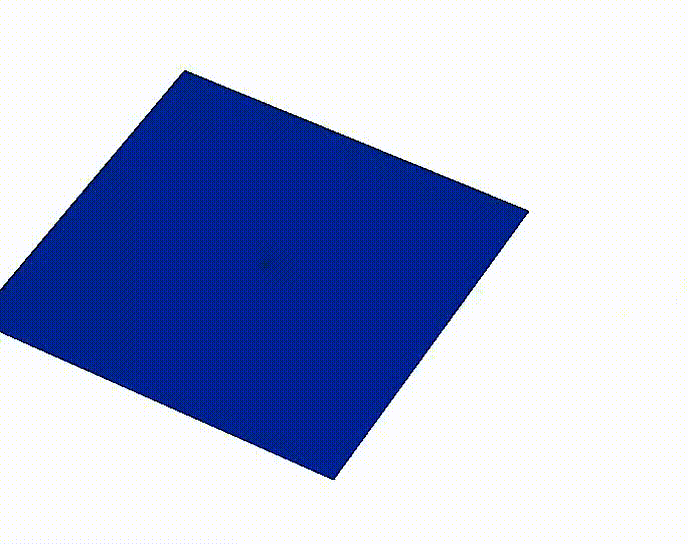# Social network analysis — Part 2

Continuing from Part 1, where I showed how to build a network from scratch. I will explain 2 common network measures: centrality & assortativity. With python module networkx, they can be calculated easily. Consider our co-authorship network, namely, each node represents one author. Note that it is a complex network with many connected components and each component is an undirected multi-graph that contains multiple edges (self-loop, in this case, is not defined or does not exist in theory). There is an edge between 2 nodes if they collaborate in 1 publication.

## Centrality

‘What characterizes an important vertex?’

For our co-authorship network, to identify the most important authors, it’s enough to calculate the following 3 different measures and assign them to each author, then sort the values in descending order.

1. Degree centrality: This is the simplest centrality measure. It’s given by the number of links attached to the node. In plain words: how many publications in total has the author published? Luckily, given its simplicity, you can even calculate in pandas dataframe: given a list of lists, count how many times each unique individual appears and sort in descending order. the higher the degree, the more important the author.
2. Betweenness centrality: For finding the individuals who influence the flow around a system. Vertices that have a high probability to occur on a randomly chosen shortest path between two randomly chosen vertices have a high betweenness. This measure shows which nodes are ‘bridges’ between nodes in a network. It does this by identifying all the shortest paths and then counting how many times each node falls on one.
3. PageRank: It uncovers nodes whose influence extends beyond their direct connections into the wider network. PageRank assigns nodes a score based on their connections, and their connections’ connections. It is similar to Eigenvector Centrality.

## Assortativity

‘Is there a tendency of nodes with the same magnitude of the degree to connect to each other, or are large-degree nodes primarily connected to low-degree nodes?’

Assortativity coefficient: It is the Pearson correlation coefficient of degree between pairs of linked nodes. The assortativity ranges from –1 to +1, positive values meaning a tendency for nodes of similar degrees to connect to each other, negative values mean that large-degree nodes tend to attach to low degree nodes. For regular graphs, this measure is not well-defined, so the function will calculate a nan value. We can interpret this as 0.

For the co-authorship network, I calculated the assortativity coefficient for each component, I then sort the values by the component size in descending order and plot it. For my partially collected data, it gives the following figure:

Since for this network, the majority of the components are of nan value, I visualized a separate graph where there are only non-null values.

As the component size decaying, the assortativity coefficient shows an asymptotic tendency to decrease. It suggests that the larger the component, the higher the probability that one author is connected with another author with similar degree. The weighted average mean of the assortativity of the whole network is 0.39, so there is a positive correlation in the general picture.

--

--

--

## More from Snow

I think, therefore, I am.

Love podcasts or audiobooks? Learn on the go with our new app.

## Data Analysis and Visualisations using R## Partitional Clustering using CLARANS method with python example## Quick reference to performance metrics of a model## Data Science## An EDA of zoo imports## The way big MNC works on collected data## How to Analyze Nominal Data## How would I learn Data Science (If I had to Start Over in 2022)## Snow

I think, therefore, I am.

## What are “Norms” in machine learning?## Prediction of Surface Roughness Using Artificial Neural Network(ANN).## Beginner’s Introduction to Natural Simulation in Python II: Simulating a Water Ripple.